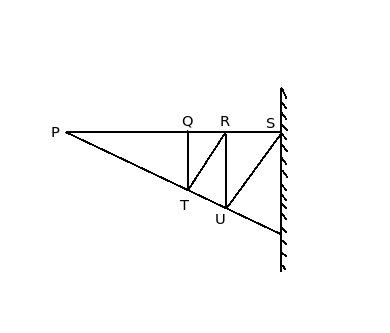# Condition for cantilever truss

Q.  Which of the following conditions is satisfied for cantilever truss?
- Published on 18 Sep 15

a. n > 2j – R
b. n < 2j – R
c. n = 2j – R
d. n ≠ 2j – R

ANSWER: n = 2j – R

#### Discussion

• Sravanthi   -Posted on 16 Dec 15
Cantilever truss: It is defined as a truss which has one end fixed and other end free.

The number of reaction components in a cantilever truss are 4, number of members are 10, joints are 7

Therefore, using the condition n = 2j – R

10 = (2 x 7) – 4 = 10

Hence, it is concluded that it is a perfect truss because the condition is satisfied.## ➨ Post your comment / Share knowledgeEnter the code shown above:

(Note: If you cannot read the numbers in the above image, reload the page to generate a new one.)